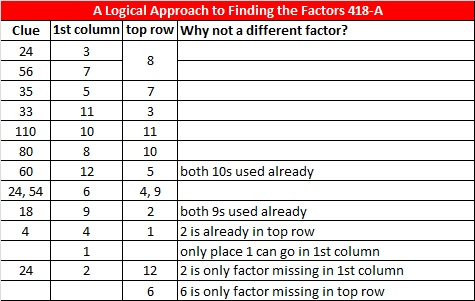# 112 and Level 4

112  is a composite number. 112 = 1 x 112, 2 x 56, 4 x 28, 7 x 16, or 8 x 14. Factors of 112: 1, 2, 4, 7, 8, 14, 16, 28, 56, 112. Prime factorization: 112 = 2 x 2 x 2 x 2 x 7, which can also be written 112 = 2⁴ x 7.112 is never a clue in the FIND THE FACTORS puzzles.

Some people get the factors of 54 and 56 mixed up. If you get them mixed up on this particular puzzle, you could still think you solved the puzzle correctly even if you haven’t. Here’s a couple of tricks that might prevent the mix-ups:

One of my favorite multiplication rhymes is “Five, Six, Seven, Eight, Fifty-Six is Seven Times Eight,” so 56 = 7 x 8.

Notice that 5 + 4 = 9. The sum of the digits of any multiple of 9 will always be 9 or another multiple of 9, so 54 = 9 x 6. On the other hand, 5 + 6 = 11, so 56 cannot have 9 as one of its factors.Excel file of six puzzles and the previous week’s solutions: 12 Factors 2014-05-05